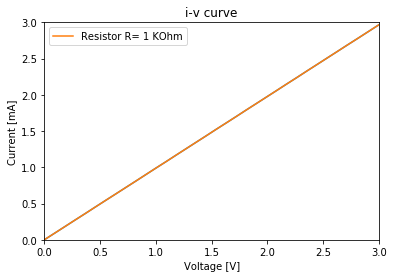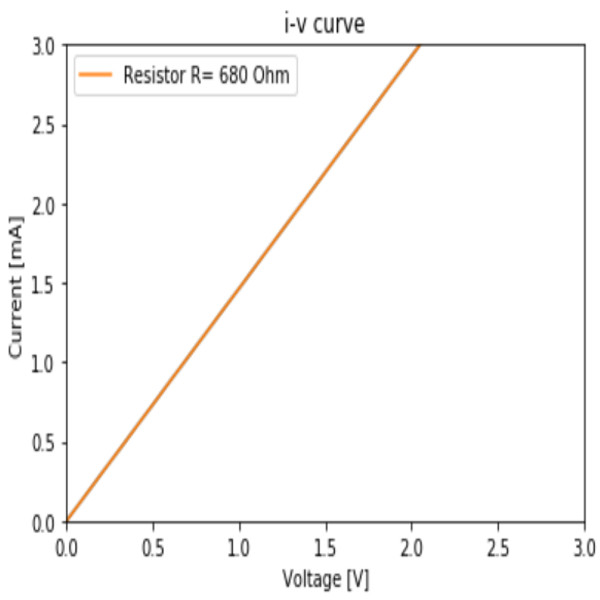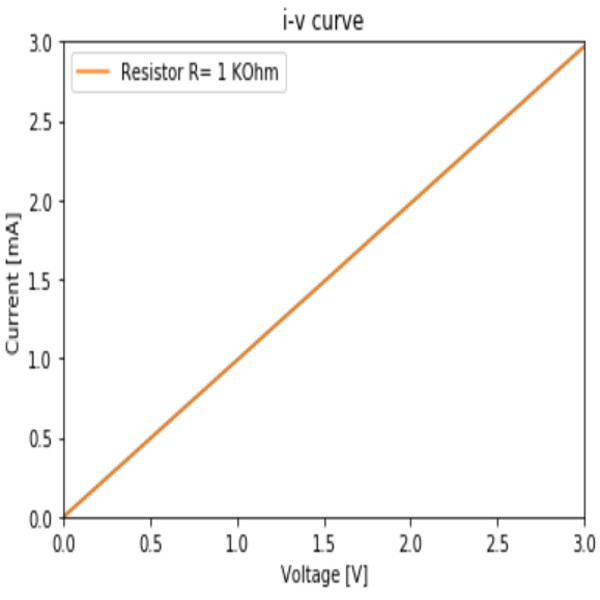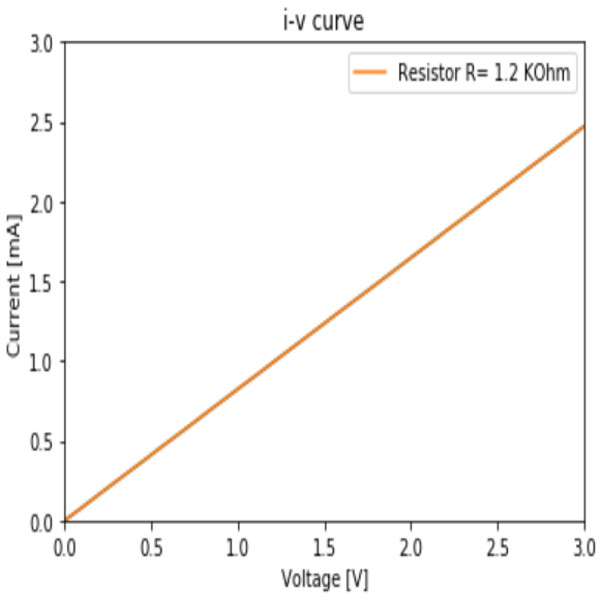Ohm Law Ohm's law states that the current through a conductor between two points is directly proportional to the voltage across the two points[wikipedia]. An I-V curve tracer is a specialised piece of electronic test equipment used to analyse the i-v characteristics of electronic components. The I-V curve tracer can be used to test various semiconductor devices such as diodes, transistors, and thyristors, however it can be also used to demonstrate Ohm's law (the linear relation between current and voltage in a resistor). The simple I-V curve tracer (picture and scheme left) was built with I2Bricks (ARD, ADC, DAC) and controlled by some Python code (below).In :
#Example Python code to demostrate ohm low.

from I2Brick import *
import matplotlib.pyplot as plt
%matplotlib inline

port = I2Brick('COM7')             #Windows: Initialize serial communication with I2Bricks
#port = I2Brick('/dev/ttyUSB0')    #Linux: Initialize serial communication with I2Bricks

print(ard(port))                   #test communication between ARD and PC

R_sense=100
voltage=[]
current=[]

for v in range (0, 4000,100):
dac(port,0, v)               #set dac voltage

port.close()

plt.plot(voltage, current)
plt.plot(voltage, current,label='Resistor R= 1 kOhm')
plt.axis([0,3,0,3])
plt.title('i-v curve')
plt.legend()
plt.xlabel('Voltage [V]')
plt.ylabel('Current [mA]')
plt.show()

I2Brick Firmware 3.0. Supported brick: ARD, OLD, DAC, ADCDCA75 Pro Advanced Semiconductor Analyser For comparison, an identical test of 1 kOhm resistor was conducted with a Peak Electronics Atlas DCA75 Pro Advanced Semiconductor Analyser (right).There are more results for the simple I2Bricks I-V curve tracer controlled by Python code below.